Trigonometric Angles
 Home > Lessons > Trigonometric Angles Search | Updated March 23rd, 2021
Introduction
In this section, you will learn how to simplify trigonometric expressions. Here are the sections within this lesson:

 Introduction to Angles Graphing trigonometric angles is fundamental to understanding all of trigonometry. It is also critical for understanding common problems that contain angles.     As we will see within the lessons below, there are many problems involving angles within several fields, like engineering, astronomy, and geography. Surveyors, pilots, landscape designers and people within many other professions heavily use angles and trigonometry to accomplish a variety of practical tasks. Standard Trigonometric Angles When we graph standard trigonometric angles we start to the East (right side of graph) and rotate counterclockwise. We can take a look at 60 degrees for our first example. The terminal end of the angle rests in quadrant I.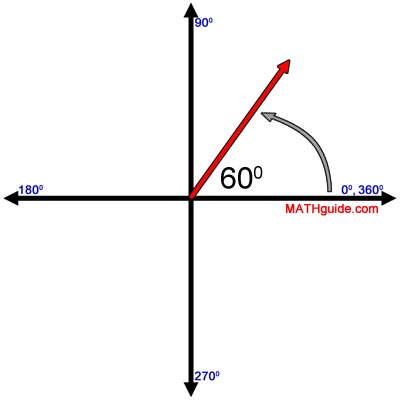Now, we will take a look at a 210 degree angle. The terminal end of this angle rests in quadrant III.Bearing Angles and Heading Angles These angles are used by pilots and the military. Instead of starting to the East, as we do for standard trigonometric angles, these angles start to the North. They also rotate clockwise instead of counter-clockwise.     For instance, a bearing of 300 degrees starts to the North and rotates clockwise, like so.A bearing of 240 degrees looks like this.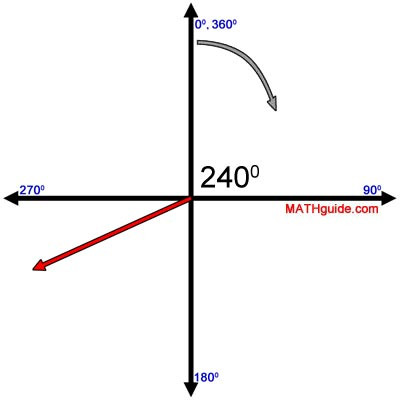Directional Angles Directional angles are used by meteorologists, pilots, and common citizens. To understand this system of angles, one has to first understand directions. Here is a picture with all the directions included.We will now look at two examples to understand this system. Example: A meteorologist says that the wind is blowing �40 degrees North of East.�     We would start with the East. Then, we would rotate 40 degrees North of that, like so.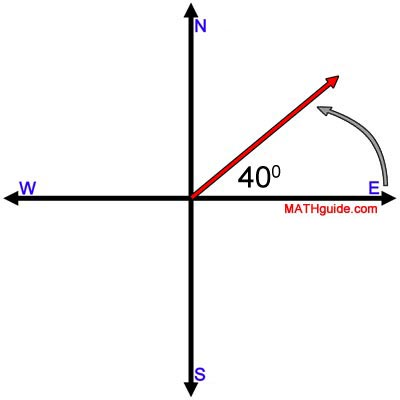Example: An air traffic controller stated that a plane is traveling �55 degrees South of West.�     First, we start to the West. Then, we rotate 55 degrees South, like so.Reference Angles A reference angle is the smallest angle measured to the x-axis. Example: Graph a 210 degree angle.     We start by graphing the angle. This is a standard angle; so, we start in the East (to the right or the positive x-axis) and then rotate counterclockwise.Next we would calculate the reference angle. 210 degrees is 30 degrees past 180, which means the reference angle is 30 degrees.Example: If we were asked to calculate the reference angle for 330 degrees, we would first sketch it.Next, we would see that it is 30 degrees from 360 degrees, which is the smallest angle to the x-axis and therefore the reference angle.Try our quizmaster to test whether or not you comprehend this lesson.ideo: Reference Anglesuiz: Calculate the Reference Angle Radians Degree measure is not the only standard for measuring an angle. Radians are a different measure for dealing with angles. Understanding radians requires visualizing a circle of radius equal to one. The circumference of this circle can be calculated using this formula, where the r-value is the radius.We will substitute the value '1' because we are working with a unit circle.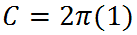Two times pi times one is 2π.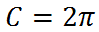This is exactly the conversion we use for dealing with 360 degrees, which is also a full circle. So, 360 degrees is equivalent to 2π.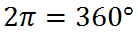When we convert from radians to degrees and degrees to radians, we will use this to do problems. Radian Conversions Now that we know radians exist, we have to be able to convert between radians and degrees.     First, we need to review how units are converted. If we wanted to convert 6 feet into inches, we have to know the conversion between those units. We know that 12 inches is equal to 1 foot. So, we will multiply 6 feet by the correct unit conversion, like so.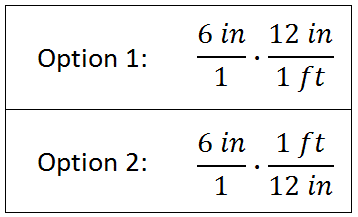Option #2 is the preferred method, because units 'cancel,' unlike option #1. Here is the work.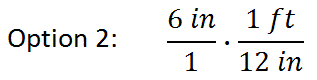Since this is the correct set-up for our problem, we will multiply.We can use the same strategy for working with all conversions, including the conversion between radians and degrees. When we work with angles, we normally think of 360 degrees and 2π, like so.So, we can form one of two conversion fractions:However, we can reduce those fractions, due to this fact: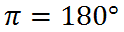This means mathematics textbooks routinely do not use 360 degrees and instead use the 180 degrees. This means we will use these conversion fractions: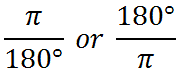We need to look at a few problems in order to understand how this conversion works. Example: Convert 120 degrees into radians.     We will take 120 degrees and multiply so that the angle measures 'cancel.'Next, we will multiply across.Lastly, we will reduce the fraction for our final answer.Try a video and a quiz to see if you can convert degrees to radians.ideo: Converting Between Degrees and Radiansuiz: Degrees to Radians Example: Convert 2/3 π into degrees.     We will multiply our radian angle so that the π 'cancels.'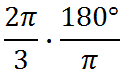Next, we will 'cancel,' and then multiply across.We will finish cleaning up to gain our final answer.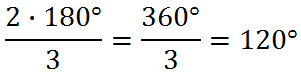Try a video and a quiz to see if you can convert radians to degrees.ideo: Converting Between Degrees and Radiansuiz: Radians to Degrees Trigonometric Functions of Special Angles Watch this video that covers this topic.ideo: Trigonometric Functions of Special Angles Inverse Trigonometric Functions Watch this video that covers this topic.ideo: Inverse Trigonometric Functions     Why are there domain restrictions on trigonometric functions?, you ask. The video below explains it.ideo: Inverse Trigonometric Functions: Domain Restrictions The Unit Circle This video will demonstrate how to use The Unit Circle to calculate ratios and angles for trigonometry problems. The unit circle is easier to use than reference angles and reference triangles. However, it is necessary to see a few examples and understand its construction to benefit from it.ideo: The Unit Circle: Trigonometryuiz: The Unit Circle: Ratiosuiz: The Unit Circle: Angles Videos Watch our instructional videos.ideo: Reference Anglesideo: Converting Between Degrees and Radiansideo: Trigonometric Functions of Special Anglesideo: Inverse Trigonometric Functionsideo: The Unit Circle: Trigonometry Quizmasters Try our interactive quizzes to determine if you understand the lessons above.uiz: Calculate the Reference Angleuiz: Degrees to Radiansuiz: Radians to Degreesuiz: The Unit Circle: Ratiosuiz: The Unit Circle: Anglesuiz: Trigonometric Angles: Find the Quadrant Related Lessons Try these lessons, which are closely related to the lessons above.esson: Trigonometry Basicsesson: Trigonometry with Right Anglesesson: Combining Vectorsesson: Sum & Difference Angle Formulas (Sine, Cosine)esson: Sum & Difference Angle Formula (Tangent)esson: Law of Sinesesson: Law of Cosinesesson: Trigonometric Expressionsesson: Polar Coordinates